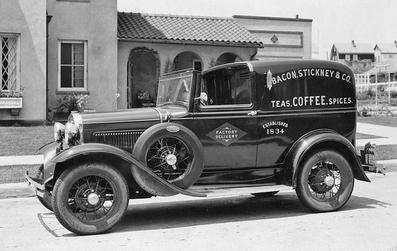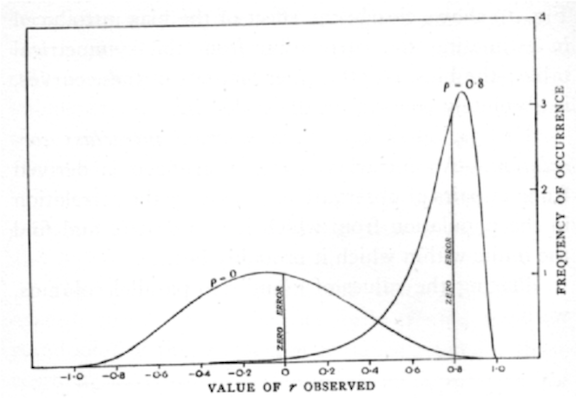## Three styles

Three different audiences in one.

1. Students, seeing data-centric lessons
2. Instructors, learning some new tools and styles
3. Budding data scientists, learning tools for doing data science

## Results from the survey

1. I am willing to take this survey. 94% (sampling bias)
2. Institution type: two thirds from two-year colleges. One from high school. Remaining from 4-year institutions.
3. Everyone responding has taught introductory statistics.

## Results from the survey

1. What coursework did we take in graduate school?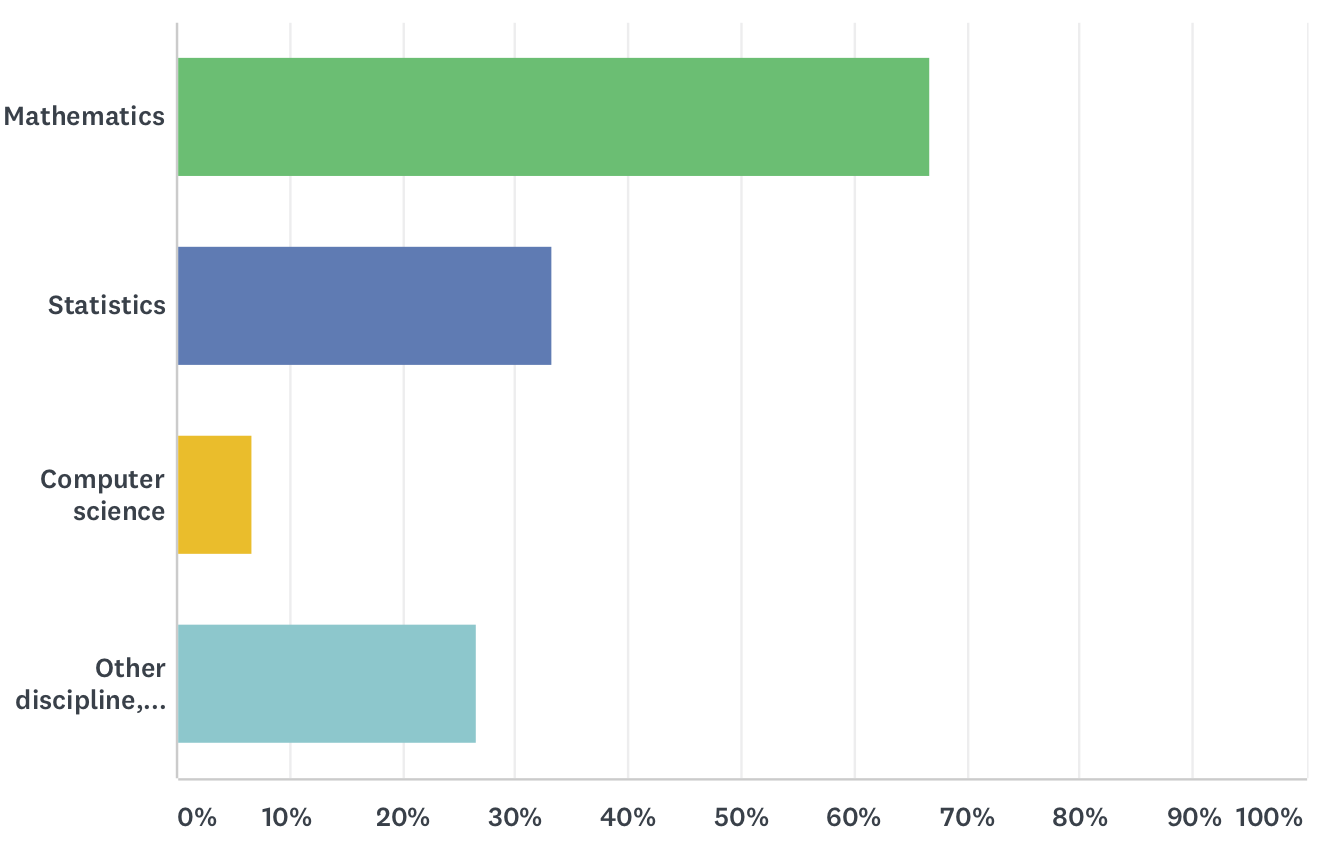## Results from the survey

1. Almost half have taken applied stats coursework.
2. Almost half "conduct statistical analyses of data outside of teaching (e.g., for your own research, consulting, etc.)"

## Results from the survey

1. Computing used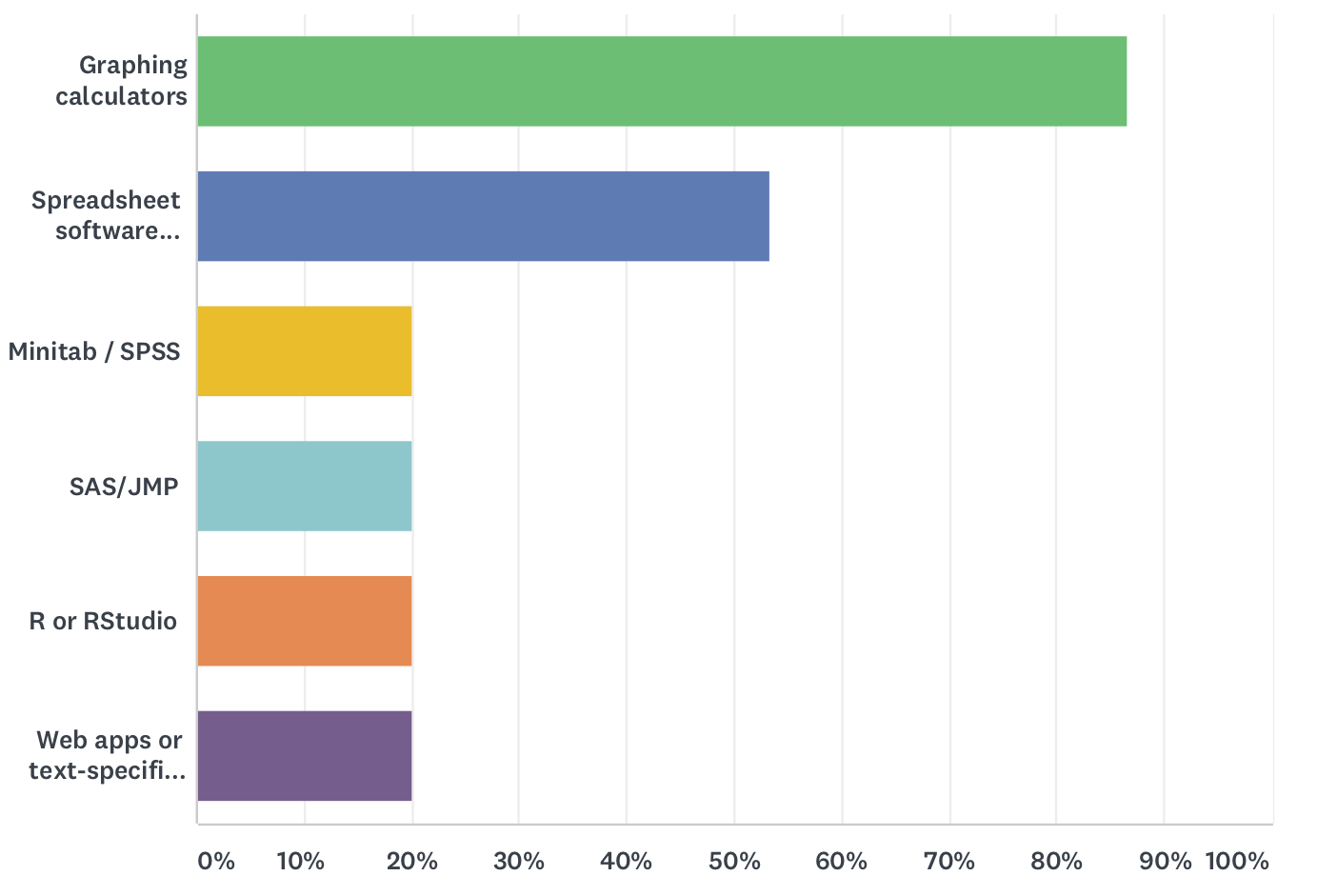## Results from the survey

1. We lack
• Personal experience handling large data sets: 55%
• Technology infrastructure for large data sets: 45%
• Graphing ability: 60% [ci at 95%: 40-80%]
2. Obstacles to making changes
• Not enough personal time: 70%
• Student characteristics: 55%
• Technology constraints: 60% [ci at 95%: 40-80%]
• Department or institutional constraints: 30%

"Too many students can't take the intro stats course, and hence graduate, because of the burden of "intermediate algebra" prerequisite."

## Results from the survey

Areas of consensus

• Students learn statistics more effectively by learning fewer topics in greater depth than learning more topics in less depth. 95% agree or strongly agree
• The many methods covered in introductory statistics can be reduced to a small set of common principles. 85% agree
• Rules of probability should be included in an introductory statistics course. 85% agree.
• Students learn statistics more effectively from a good lecture than from a good activity. 80% disagree or strongly disaggree

## Results from the survey

More areas of concesus

• Algebraic formulas are the best method to learn statistics. 100% disagree
• Students learn statistics better by understanding how to express concepts with algebra. 85% disagree
• Computing offers a framework for understanding statistical theory that is as legitimate as the theory based on probability rules and algebra. 80% agree
• Students should learn how to read statistical tables of theoretical distributions (e.g., t-table, F-table). 80% disagree

GAISE College report (p. 24)

Drills with z-, t-, χ2, and F-tables. These skills are no longer necessary and do not reflect modern statistical practice. Since statistical software produces a p-value as part of performing a hypothesis test, a shift from finding p-values to interpreting p-values in context is appropriate.

## p value quiz

ASA statement:

Underpinning many published scientific conclusions is the concept of “statistical significance,” typically assessed with an index called the p-value. While the p-value can be a useful statistical measure, it is commonly misused and misinterpreted.

## Quiz: Agree or disagree?

1. P-values can indicate how incompatible the data are with a specified statistical model.

2. P-values do not measure the probability that the studied hypothesis is true, or the probability that the data were produced by random chance alone.

3. A p-value, or statistical significance, does not measure the size of an effect or the importance of a result.

4. By itself, a p-value does not provide a good measure of evidence regarding a model or hypothesis.

## Theory or practice?

Is statistics …

• an area of theory (like group theory or number theory or graph theory)?
• a practical tool?

Insofar as statistics is a practical tool, the stat education community some catching up to do.

## 1880s Galton and Benz

• 1885 Karl Benz designs 4-stroke engine for use in his automobile
• 1888 Francis Galton introduces the "co-relation" coefficient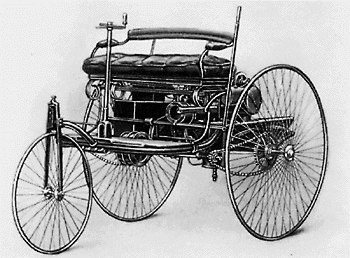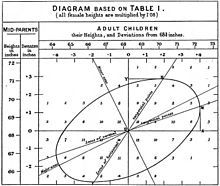## 1908 Gossett and Ford

• First Model T off Henry Ford's production line
• William Gossett's t statistic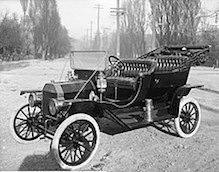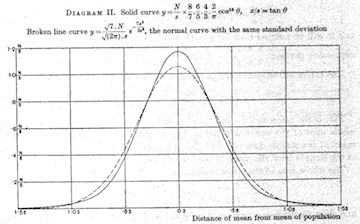## 1920s

• 1925 ANOVA appears in Fisher's Statistical Methods for Research Workers
• 1927 Ford Model A enters production